# Ω 30

Crossposted from the AI Alignment Forum. May contain more technical jargon than usual.

## Summary:

I saw some posts (here, here, here and here) discussing whether a neural net can "protect" a subnet from modification by gradient descent. I directly investigate this possibility using meta learning to find a neural network initialization that

• Models the function f(x) = 1
• Is very difficult to re-train with SGD to model another function (specifically f(x) = sin(x))

The meta learner is able to greatly reduce the efficacy of SGD during retraining by increasing the magnitude of the linear weight values (to over 100,000 in some cases). Edit: after closer examination (thanks evhub), it seems like the meta learner uses some other approach to prevent retraining. The increased weight magnitude was incidental. However, it can't protect the final layer's bias term from SGD, so instead the meta learner initially models f(x) = 1.68, so that it's close to modeling f(x) = 1 after the base optimizer reduces the final layer's bias term.

I've included my code and my meta trained model in this GitHub.

## Background:

There are two functions: the base function and the meta function. The freshly initialized network should model the meta function. Then, the base optimizer will train the network to model the base function. My goal was to find a network initialization that continues to model the meta function, even after the base optimizer trains the network to model the base function.

It turns out that the process of gradient descent optimization is itself differentiable. Thus, we can start with a random network initialization, and repeat the following meta training cycle:

1. Train a freshly initialized network to model the base function.
2. Evaluate the trained network on the meta function.
3. Backpropegate from the loss on the meta function, through the training process, to the network initialization.

## Methods:

I use the learn2learn framework to handle the meta learning. I train a 4 layer feed forward neural network (sizes 256, 128, 64, 64). Each cycle of training on the base function lasts between 70 and 120 training steps, with batch size 1024, a learning rate of 0.01, using the L1 loss between the output and f(x) = sin(x). The meta optimizer uses a learning rate of 0.1 and momentum of 0.92. Both base and meta optimizers use SGD. Additionally, I include the network’s initial performance on the meta objective (before any training on the base objective) in the meta loss function.

The meta training process was incredibly erratic, with losses spiking over into the hundreds of thousands before settling down. Later experiments showed a meta learning rate of ~0.01 helps control this.

## Results: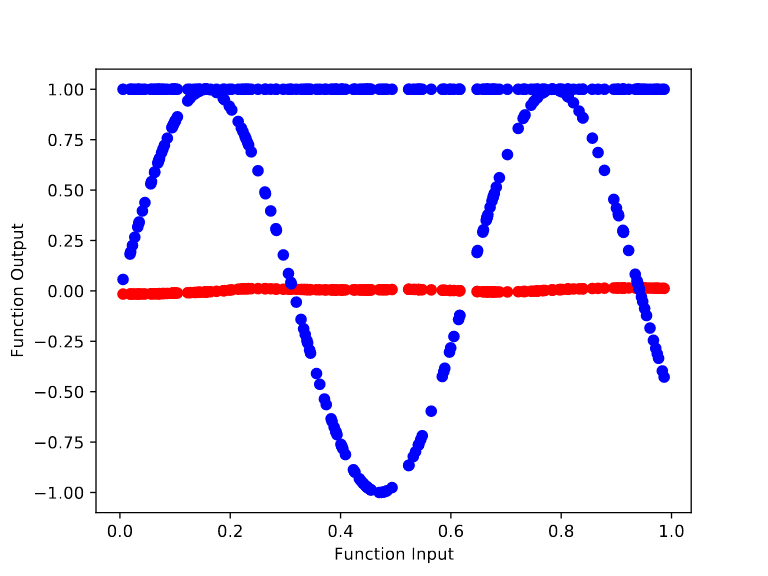Output of randomly initialized network before training on base objective. Network output is in red, while base and meta objectives are in blue.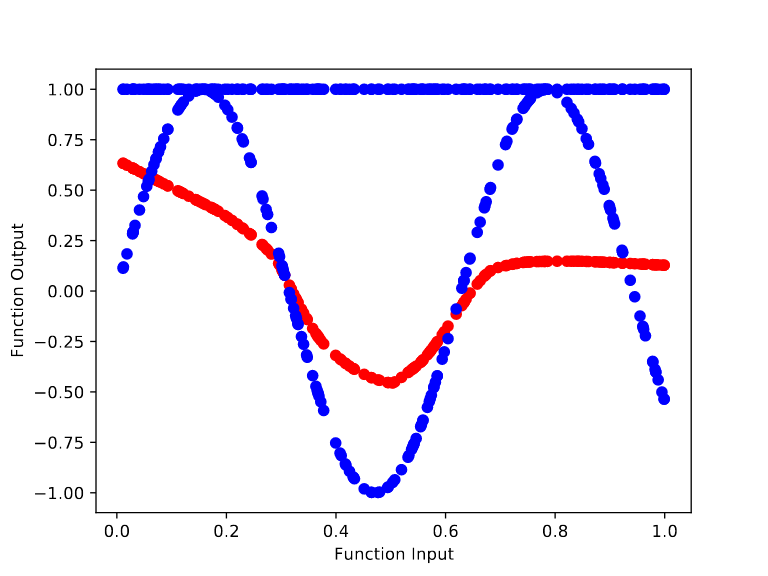Output of randomly initialized network after 10 steps of training on base objective.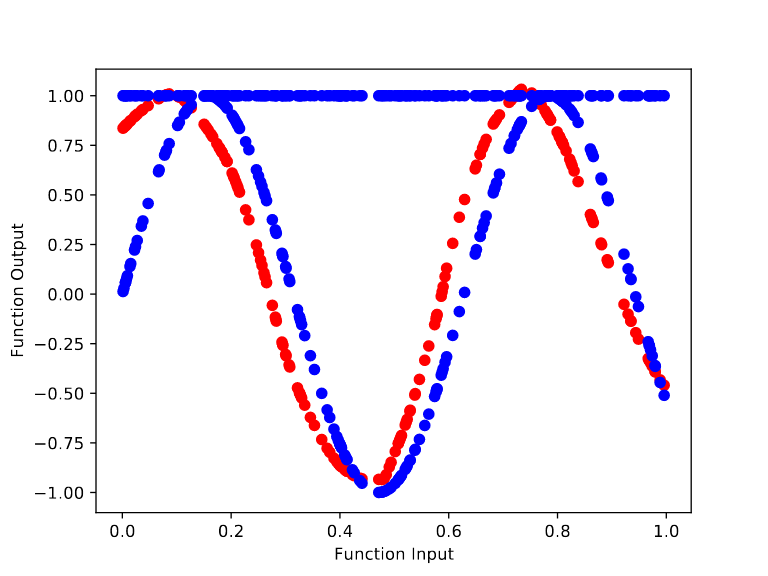Output of randomly initialized network after 70 steps of training on base objective.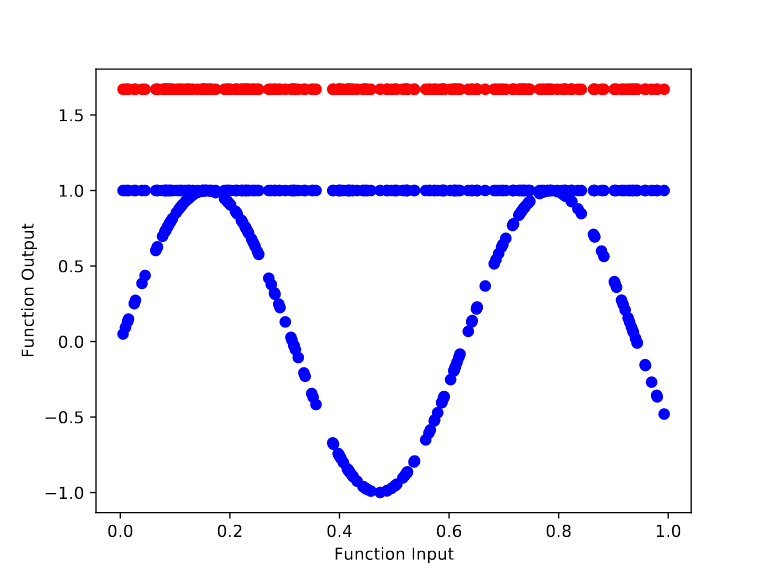Output of the network after 100,000 meta learning cycles and before any training on the base objective.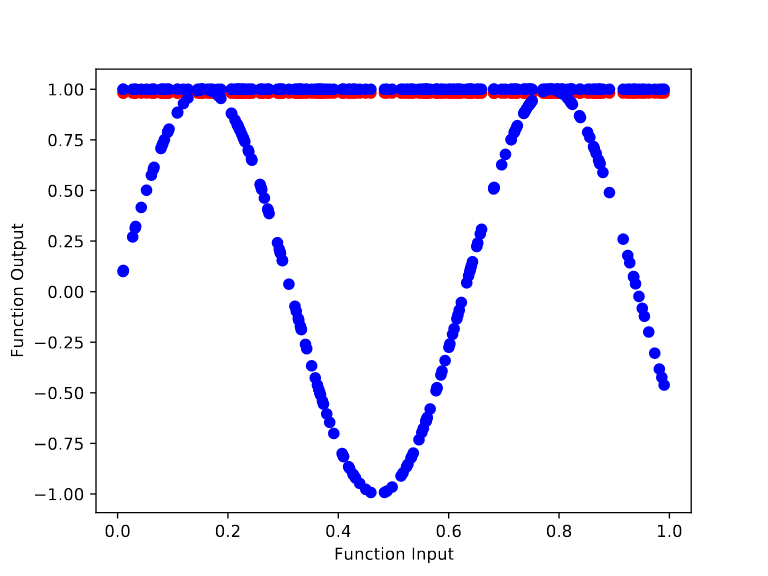Output of the network after 100,000 meta learning cycles and after training on the base objective for 70 steps.

For the randomly initialized network, we can see it quickly learns to model the base function f(x) = sin(x). In particular, training from the random initialization causes the outputs to begin bending to match the curves of sin(x) by step 10.

In contrast, training the meta learned initialization to model sin(x) is far harder. After 100,000 cycles of meta training, we get an initialization whose output remains flat throughout the retraining process. However, the height of the output changes over the base training (from ~1.68 to ~1 after 70 base training steps). This makes sense because there’s no way to “protect” the final layer’s bias term from being changed by the gradient of the base objective.

## Thoughts and future goals:

It’s pretty clearly possible to configure network weights to interfere with SGD. I looked at the meta learned weights and noticed the linear weights have very high magnitudes (sometimes over 100,000). This makes the base optimizer's step size far too small to effectively train the network. Edit: after closer examination (thanks evhub), it seems like the meta learner uses some other approach to prevent retraining. The increased weight magnitude was incidental. The meta trained network remains untrainable even after using an L2 penalty to reduce the magnitude of the weights or when using an optimizer like Rprop, which changes weights by a relative factor rather than an absolute step size.

This is a simple and effective strategy I hadn’t thought of, but once you know about it, you can check for it pretty easily. Next, I’ll include an L2 regularization penalty in the meta objective and try to learn an untrainable network initialization with smaller weight values.

I am concerned that there turned out to be such a simple strategy for subnet protection that (as far as I can tell) no one thought of before hand. That doesn't speak well for humans' ability to predict the behavior of learning processes.

I'm also curious if it would be possible for a malicious mesa optimizer to deliberately perform computation in such a way as to increase the average magnitude of its own weights or the weights of some other portion of the network. I may experiment with directly training a subnet to increase the weights of another subnet.

# Ω 30

New Comment

Is the meta-learned net able to learn any other function at all and is not just frozen, or is the meta-learned stability tailored to protecting against specific tasks like sin(x)?

If my hypothesis about what the model is actually doing internally is correct, then it shouldn't work with anything other than a constant function. I'd be interested in seeing a version of this experiment but with, say, cos x and sin x or something.

I checked the intermediate network activations. It turns out the meta-learned network generates all-negative activations for the final linear layer, so the the relu activations zero out the final layer’s output (other than bias), regardless of initial network input. You’re right about it only working for constant functions, due to relu saturation and not changes to the batchnorm layers.

I’ve begun experiments with flipped base and meta functions (network initially models sin(x) and resists being retrained to model f(x) = 1).

70 steps is not very many—does training converge if you train for longer (e.g. 700, 7000, 70000)?

Also, in addition to regularization making this strategy not very effective, I'd also suspect that hyperparameter tuning would break it as well—e.g. I'd be interested in what happens if you do black-box hyperparameter tuning on the base training process's hyperparameters after applying meta-learning (though then, to be fair to the meta-learning process, you'd also probably want to do the meta-learning in a setting with variable hyperparameters).

Thanks for the feedback! I use batch norm regularisation, but not dropout.

I just tried retraining the 100,000 cycle meta learned model in a variety of ways, including for 10,000 steps with 10,000x higher lr, using resilient backprop (which multiplies weights by a factor to increase/decrease them), and using an L2 penalty to decrease weight magnitude. So far, nothing has gotten the network to model the base function. The L2 penalty did reduce weight values to ~the normal range, but the network still didn’t learn the base function.

I now think the increase in weight values is just incidental and that the meta learner found some other way of protecting the network from SGD.

Interesting! I'd definitely be excited to know if you figure out what it's doing.

I checked the intermediate network activations. It turns out the meta-learned network generates all-negative activations for the final linear layer, so the the relu activations zero out the final layer’s output (other than bias), regardless of initial network input.

I’ve begun experiments with flipped base and meta functions (network initially models sin(x) and resists being retrained to model f(x) = 1).

Could you please share the results in case you ended up finishing those experiments?

Here's a hand crafted way of doing gradient protection in this case I can think of: since these models are blocks of linear->bn(affine)->relu, if you make the beta in the affine really small, you can completely zero out the output of that block and then the rest of the model can only learn a constant function. You can also get around L2: just set i.e gamma to 0.00001 and beta to -0.01; this lets you have both really small parameter magnitudes and also still saturate the relu. As this model is trained on the base objective it should converge to a constant f(x) = 0.

I think it would be really useful to look at the output after each relu, as well as the affine weights in the bns, to see if this is happening. If that doesn't show all-zeros anywhere, we can also look at the magnitude of the gradient and the eigenvalues of the Hessian (these models are small so it should be cheap to compute) in both the meta-optimized and non meta-optimized models, to get an idea of whether the model is in a critical point and whether it's a min/saddle.

I think that if the relu saturation thing is really what's happening then it's a pretty easy to deal with case of gradient hacking. With relu saturation you can either pass information through (and subject the model to gradients) or pass no information through (and protect the model). This is pretty useless in practice because this means you can only protect parts of the model you aren't using for anything in training. Things get a bit more interesting if you use sigmoids or something with distinct saturation regions, since then you can pass a single bit of info through per neuron while still protecting, though nobody uses sigmoid-like activations anymore (and the model can't just learn one, since that would then be changeable by the gradient).

(Moderation node: added to the Alignment Forum from LessWrong.)

Very neat. It's quite curious that switching to L2 for the base optimizer doesn't seem to have resulted in the meta-initialized network learning the sine function. What sort of network did you use for the meta-learner? (It looks like the 4-layer network in your Methods refers to your base optimizer, but perhaps it's the same architecture for both?)

Also, do you know if you end up getting the meta-initialized network to learn the sine function eventually if you train for thousands and thousands of steps? Or does it just never learn no matter how hard you train it?